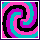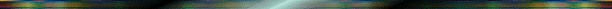## Mathematical Construction of Spirals### These techniques are simple. All they require are graph paper, pencil, and either a steady hand and a good eye, or a drafting curve to help you connect the dots you put on the graph paper. We could do this on ordinary rectangular graph paper (Cartesian coordinate system), or on circular graph paper (polar coordinate system). But because we think of the spiral as created by increasing the distance from the center to the outside of a circle as we go around the circle, using the polar coordinate system is more like the way we actually think of drawing the spiral. It also avoids trigonometry that we need to use the rectangular coordinate system accurately. The Polar Coordinate System The circular graphic method Making the Spirals The geometric techniques Difference Equations The dynamical math used Definitions Pattern, spiral, difference equation Exercises Answers to Exercises 1-12.Created: 12/19/96 Updated: 12/25/96